Courses

# Optical Instruments JEE Notes | EduRev

## JEE : Optical Instruments JEE Notes | EduRev

The document Optical Instruments JEE Notes | EduRev is a part of the JEE Course HC Verma and Irodov Solutions.
All you need of JEE at this link: JEE

Page-430

Ques 1:Can virtual image be formed on the retina in a seeing process?

Ans:The retina acts as a screen; only real images can be obtained on the screen. In case of people having eye defects, the spectacles form the virtual image of the object and the eye lens form the real and inverted image on the retina.

Ques 2:Can the image formed by a simple microscope be projected on a screen without using any additional lens or mirror?

Ans:The image formed by a simple microscope is virtual and erect. So, it cannot be projected on a screen without using any additional lens or mirror.

Ques 3:The angular magnification of a system is less than one. Does it mean that the image formed is inverted?

Ans:No, angular magnification is the ratio of the angle subtended by the final image on the eye to the angle subtended by the object on the unaided eye. Its value less than one signifies reduction in the size of the image. It does not mean that the image is inverted.

Ques 4:A simple microscope using a single lens often shows coloured image of a white source. Why?

Ans: A simple microscope consists of a single convex lens. Sometimes due to chromatic and spherical aberrations, the image of a white source seems coloured at the corners of the lens and somewhere in between.

Ques 5: A magnifying glass is a converging lens placed close to the eye. A farsighted person uses spectacles having converging lenses. Compare the functions of a converging lens used as a magnifying glass and as spectacles.

Ans: A converging lens in a magnifying glass is of small focal length which is used to magnify an object which is placed close to the lens. On the other hand, converging lens used as spectacles is of varying focal length which depends upon the actual near point of the long-sighted person. It forms image at the near point of the defected eye which is further focussed by the eye lens.

Ques 6:A person is viewing an extended object. If a converging lens is placed in front of his eyes, will he feel that the size has increased?

Ans: If a person views an extended object through a converging lens, then it will appear larger and wider to him.

Ques 7:The magnifying power of a converging lens used as a simple microscope is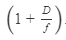A compound microscope is a combination of two such converging lenses. Why don't we have magnifying power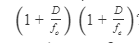In other words, why can the objective not be treated as a simple microscope but the eyepiece can?

Ans:In a simple microscope, the converging lens is used to magnify the object. It is done by the eyepiece in a compound microscope. But the purpose of the objective lens is the same, i.e., to form an enlarged, real and inverted image of the object at a distance less than the focal length of the eyepiece. So, its magnification power cannot be expressed in a way it is expressed for a simple microscope.

Ques 8:By mistake, an eye surgeon puts a concave lens in place of the lens in the eye after a cataract operation. Will the patient be able to see clearly any object placed at any distance?

Ans:The image formed by a concave lens is virtual and upright. It is smaller than the object and is formed between the object and the lens, irrespective of the position of the object. If, by mistake, an eye surgeon puts a concave lens in place of eye lens in a patient's eye, then the image will not be focused on the retina; this will lead to unclear vision.

Question 9:The magnifying power of a simple microscope is given by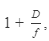where D is the least distance for clear vision. For farsighted persons, D is greater than the usual. Does it mean that the magnifying power of a simple microscope is greater for a farsighted person as compared to a normal person? Does it mean that a farsighted person can see an insect more clearly under a microscope than a normal person?

Ans:The magnifying power of a simple microscope depends on the ratio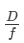for a farsighted person. Here, D for a farsighted person is greater than that for a normal person, but the value of f remains the same. Therefore, the magnifying power of a simple microscope is greater for a farsighted person compared to that for a person with normal vision. Also, a farsighted person can see the insect more clearly under the microscope than a person with normal vision.

Ques:Why are the magnification properties of microscopes and telescopes defined in terms of the ratio of angles and not in terms of the ratio of sizes of objects and images?

Ans:Instruments like telescopes and microscopes deal with objects placed at different distances. Due to some physical factors, there is a relative change in heights not in the angle which the light emerging from them subtends on the lens. So, the magnification properties of instruments are defined in terms of the ratio of angles.

Ques 11:An object is placed at a distance of 30 cm from a converging lens of focal length 15 cm. A normal eye (near point 25 cm, far point infinity) is placed close to the lens on the other side. (a) Can the eye see the object clearly? (b) What should be the minimum separation between the lens and the eye so that the eye can clearly see the object? (c) Can a diverging lens, placed in contact with the converging lens, help in seeing the object clearly when the eye is close to the lens?

Answer 11:

Object distance, u =-30 cm

Focal length, f = 15 cm

Image distance, v = ?

The lens formula is given by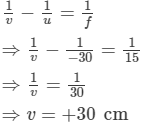(on the opposite side of the object)

(a) No, the eye placed close to the lens cannot see the object clearly.

(b) The eye should be 30 cm away from the lens to see the object clearly.

(c) The diverging lens will always form an image at a large distance from the eye; this image cannot be seen through the human eye.

Ques 12:A compound microscope forms an inverted image of an object. In which of the following cases it it likely to create difficulties? (a) Looking at small germs. (b) Looking at circular spots. (c) Looking at a vertical tube containing some water.

Ans:(c) Looking at a vertical tube containing some water

If the experimentalist is looking at a vertical tube containing some water, he has to be careful, as the lower meniscus will appear as upper.

Page-431
OBJECTIVE-1

Ques 1:The size of an object as perceived by an eye depends primarily on

(a) actual size of the object

(b) distance of the object from the eye

(c) aperture of the pupil

(d) size of the image formed on the retina.
Ans: (d) size of the image formed on the retina

An eye consists of a lens that works on the principle on which a glass lens works. It forms the image on the screen called retina. The magnification, in this case, depends on the ratio of the image to the object height.

Que 2:The muscles of a normal eye are least strained when the eye is focussed on an object

(a) far away from the eye

(b) very close to the eye

(c) at about 25 cm from the eye

(d) at about 1 m from the eye

Ans:(a) far away from the eye

A normal eye can see from 25 cm to infinity; it faces least difficulty and strain focussing on the object as far as it could be.

Ques 3:A normal eye is not able to see objects closer than 25 cm because

(a) the focal length of the eye is 25 cm

(b) the distance of the retina from the eye-lens is 25 cm

(c) the eye is not able to decrease the distance between the eye-lens and the retina beyond a limit

(d) the eye is not able to decrease the focal length beyond a limit.

Ans:(d) the eye is not able to decrease the focal length beyond a limit

The ciliary muscles adjust the focal length to form an image on the retina, but the muscles cannot be strained beyond a limit. Hence, if the object is brought too close to the eye, the focal length cannot be adjusted to form the image on the retina.

Ques 4:When objects at different distances are seen by the eye, which of the following remain constant?

(a) The focal length of the eye-lens.

(b) The object-distance from the eye-lens.

(c) The radii of curvature of the eye-lens.

(d) The image-distance from the eye-lens.

Ans:(d) The image distance from the eye lens

In the human eye, the image is formed on the retina, which is at a fixed distance from the eye lens.

Ques 5: A person A can clearly see objects between 25 cm and 200 cm. Which of the following may represent the range of clear vision for a person B having muscles stronger than A, but all other parameters of eye identical to that of A?

(a) 25 cm to 200 cm

(b) 18 cm to 200 cm

(c) 25 cm to 300 cm

(d) 18 cm to 300 cm

Ans: (b) 18 cm to 200 cm

Person B has stronger ciliary muscles than person A. So, the muscles in his case can be strained more and the focal length of his eye can be reduced more compared to those of person A. While seeing far objects, the muscles are relaxed, so their strength will not affect the far point of the eye.

Ques 6:The focal length of a normal eye-lens is about

(a) 1 mm

(b) 2 cm

(c) 25 cm

(d) 1m

Ans:(b) 2 cm

Given:

Near point of the human eye, u = -25 cm

Distance between the retina and the eye lens, v = 2 cm (approximately)

Thus, we have the focal length, f.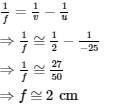Ques 7:The distance of the eye-lens from the retina is x. For a normal eye, the maximum focal length of the eye-lens

(a) = x

(b) < x

(c) > x

(d) = 2 x

Ans: (a) = x

For a normal eye, we have:

Far point at which the object can be placed, u =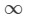Distance between the eye lens and the retina, v = x

Thus, we have: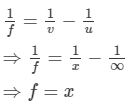Ques 8:A man wearing glasses of focal length +1 m cannot clearly see beyond 1 m

(a) if he is farsighted

(b) if he is nearsighted

(c) if his vision is normal

(d) in each of these cases

Ans:(d) in each of these cases

The man is wearing glasses of positive power (converging lens). Hence, he cannot see nearby objects clearly. In other words, he is farsighted. Since he cannot see beyond 1 m, he is nearsighted. If a person with normal vision wears glasses of focal length +1 m, then the person will not be able to see beyond 1 m.

Ques 9:An object is placed at a distance u from a simple microscope of focal length f. The angular magnification obtained depends

(a) on f but not on u

(b) on u but not on f

(c) on f as well as u

(d) neither on f nor on u

Ans:(c) on f as well as u

The angular magnification is the ratio of the angle subtended by the image to the angle subtended by the object on an unaided eye.In a simple microscope,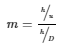Here,
u=Object distance from the lens

D=Image distance from the lens

h=Height of the object

In normal adjustment, the object is placed at a distance equal to  focal length(f)  from the lens and then magnification is given by m = D/f.

For u < f, m = D/f +1.

Ques 10:To increase the angular magnification of a simple microscope, one should increase

(a) the focal length of the lens

(b) the power of the lens

(c) the aperture of the lens

(d) the object size

Ans:(b) the power of the lens

For a simple microscope in normal adjustment, the object is placed at a distance equal to  f (the focal length) from the lens, And the angular magnification is given by the relation

m = D/f.

For u < f, m = D/f  + 1.

Power of the lens = 1/f

Angular magnification depends on power.

Ques 11:A man is looking at a small object placed at his near point. Without altering the position of his eye or the object, he puts a simple microscope of magnifying power 5 X before his eyes. The angular magnification achieved is

(a) 5

(b) 2.5

(c) 1

(d) 0.2

Ans:(c) 1

We have :
h=Object height

u=Object distance= 25 cm

D=Near point=25 cm

Now,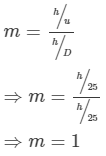OBJECTIVE-2

Ques 1:When we see an object, the image formed on the retina is

(a) real

(b) virtual

(c) erect

(d) inverted

Ans:(a) real
(d) inverted

The retina acts as a screen in the eye; only real, inverted images can be obtained on the screen.

Ques 2:In which of the following the final image is erect?

(a) Simple microscope

(b) Compound microscope

(c) Astronomical telescope

(d) Galilean telescope

Ans:(a) Simple microscope

(d) Galilean telescope

In a simple microscope, the image formed is virtual and above the axis on the same side of the object. Similarly, in a Galilean telescope, the image formed is virtual, erect and magnified and between the objective lens and the eyepiece. A compound microscope and an astronomical telescope produce inverted images.

Ques 3:The maximum focal length of the eye-lens of a person is greater than its distance from the retina. The eye is

(a) always strained in looking at an object

(b) strained for objects at large distances only

(c) strained for objects at short distances only

(d) unstrained for all distances

Ans 3:(a) always strained in looking at an object

The maximum focal length of a normal eye is equal to the distance of the lens from the retina. In case it is greater than the distance, the eye will be strained while focusing the objects on the retina that is at a fixed distance from the eye lens.

Ques 4:Mark the correct options.

(a) If the far point goes ahead, the power of the divergent lens should be reduced.

(b) If the near point goes ahead, the power of the convergent lens should be reduced.

(c) If the far point is 1 m away from the eye, divergent lens should be used.

(d) If the near point is 1 m away from the eye, divergent lens should be used.

Ans:(a) If the far point goes ahead, the power of the divergent lens should be reduced.

(c) If the far point is 1 m away from the eye, the divergent lens should be used.

As the far point (x) is shifted ahead, the focal length (f) will be increased.

Thus, we have: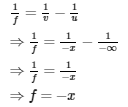As power (P) is equal to the reciprocal of the focal length, it will be reduced. Also, because the focal length is negative, the lens used will be divergent when the far point is 1 m away.

Ques 5:The focal length of the objective of a compound microscope if fo and its distance from the eyepiece is L. The object is placed at a distance u from the objective. For proper working of the instrument,

(a) L < u

(b) L > u

(c) fo < L < 2fo

(d) L > 2fo

Ans:(b) L > u

(d) L > 2f0

In a compound microscope, the objective lens of a short focal length, fo, is used. The focal length of the objective lens is less than the focal length of the eyepiece, fe, .The object is placed at a distance slightly greater than its focal length. The real, inverted image of the object forms somewhere in front of the eyepiece at a distance less than its focal length. This image acts as its object and the final image forms in between length, L, of the microscope. Please check and correct.

∴ L > fo+ fe > 2fo

Also,

L > u

Offer running on EduRev: Apply code STAYHOME200 to get INR 200 off on our premium plan EduRev Infinity!

76 docs

,

,

,

,

,

,

,

,

,

,

,

,

,

,

,

,

,

,

,

,

,

;